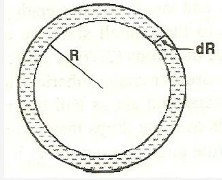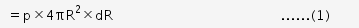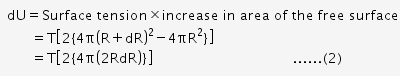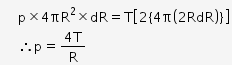# Derive an expression for excess of pressure inside a soap bubble

Derive an expression for excess of pressure inside a soap bubble.Consider a soap bubble of radius R and surface tension T. There are two free surfaces of soap bubble. Due to surface tension, the molecules on the surface film experience the net force in the inward direction normal to the surface. Therefore, there is more pressure inside than outside.

Let pi be the pressure inside the liquid drop and po be the pressure outside the drop. Therefore, excess pressure inside the liquid drop is

p = pi - po

Due to excess pressure inside the liquid drop, the free surface of the drop will experience the net force in the outward direction due to which the drop will expand.

Let the free surfaces be displaced by dR under isothermal conditions. Therefore, excess pressure does the work in displacing the surface and that work will be stored in the form of potential energy.

The work done by excess pressure in displacing the surface is
dW = force × displacement
= (excess pressure x Surface area × displacement of surface)Increase in potential energy isFrom (1) and (2), we get